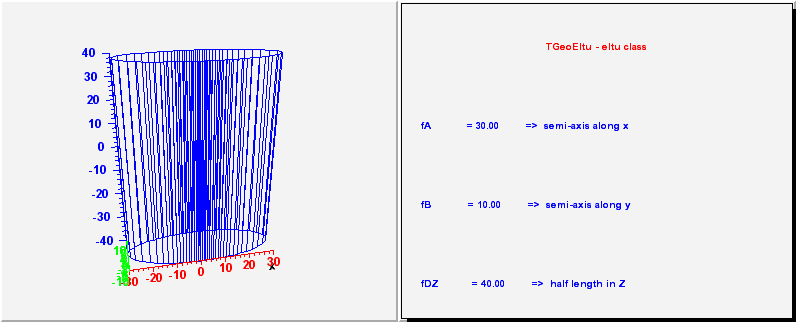library: libGeom#include "TGeoEltu.h"

# TGeoEltu

class description - source file - inheritance tree (.pdf)

## class TGeoEltu : public TGeoTube

Inheritance Chart:
 TObject
<-
TNamed
<-
TGeoShape
<-
TGeoBBox
<-
TGeoTube
<-
TGeoEltu
```
public:
TGeoEltu()
TGeoEltu(Double_t a, Double_t b, Double_t dz)
TGeoEltu(const char* name, Double_t a, Double_t b, Double_t dz)
TGeoEltu(Double_t* params)
TGeoEltu(const TGeoEltu&)
virtual ~TGeoEltu()
static TClass* Class()
virtual void ComputeBBox()
virtual void ComputeNormal(Double_t* point, Double_t* dir, Double_t* norm)
virtual Bool_t Contains(Double_t* point) const
virtual Int_t DistancetoPrimitive(Int_t px, Int_t py)
virtual Double_t DistFromInside(Double_t* point, Double_t* dir, Int_t iact = 1, Double_t step = TGeoShape::Big(), Double_t* safe = 0) const
virtual Double_t DistFromOutside(Double_t* point, Double_t* dir, Int_t iact = 1, Double_t step = TGeoShape::Big(), Double_t* safe = 0) const
virtual TGeoVolume* Divide(TGeoVolume* voldiv, const char* divname, Int_t iaxis, Int_t ndiv, Double_t start, Double_t step)
virtual Double_t GetA() const
virtual Double_t GetB() const
virtual void GetBoundingCylinder(Double_t* param) const
virtual const TBuffer3D& GetBuffer3D(Int_t reqSections, Bool_t localFrame) const
virtual TGeoShape* GetMakeRuntimeShape(TGeoShape* mother, TGeoMatrix* mat) const
virtual Int_t GetNmeshVertices() const
virtual void InspectShape() const
virtual TClass* IsA() const
virtual Bool_t IsCylType() const
TGeoEltu& operator=(const TGeoEltu&)
virtual Double_t Safety(Double_t* point, Bool_t in = kTRUE) const
virtual void SavePrimitive(ofstream& out, Option_t* option)
virtual void SetDimensions(Double_t* param)
void SetEltuDimensions(Double_t a, Double_t b, Double_t dz)
virtual void SetPoints(Double_t* points) const
virtual void SetPoints(Float_t* points) const
virtual void ShowMembers(TMemberInspector& insp, char* parent)
virtual void Streamer(TBuffer& b)
void StreamerNVirtual(TBuffer& b)
```

## Class Description

``` TGeoEltu - elliptical tube class. It takes 3 parameters :
semi-axis of the ellipse along x, semi-asix of the ellipse along y
and half-length dz.

_____________________________________________________________________________
```
/**/

TGeoEltu()
``` Dummy constructor
```

TGeoEltu(Double_t a, Double_t b, Double_t dz) :TGeoTube()
``` Default constructor specifying X and Y semiaxis length
```

TGeoEltu(const char *name, Double_t a, Double_t b, Double_t dz) :TGeoTube()
``` Default constructor specifying X and Y semiaxis length
```

TGeoEltu(Double_t *param)
``` Default constructor specifying minimum and maximum radius
param =  A
param =  B
param = dz
```

~TGeoEltu()
``` destructor
```

void ComputeBBox()
``` compute bounding box of the tube
```

void ComputeNormal(Double_t *point, Double_t *dir, Double_t *norm)
``` Compute normal to closest surface from POINT.
```

Bool_t Contains(Double_t *point) const
``` test if point is inside the elliptical tube
```

Int_t DistancetoPrimitive(Int_t px, Int_t py)
``` compute closest distance from point px,py to each vertex
```

Double_t DistFromInside(Double_t *point, Double_t *dir, Int_t iact, Double_t step, Double_t *safe) const
``` compute distance from inside point to surface of the tube
```

Double_t DistFromOutside(Double_t *point, Double_t *dir, Int_t iact, Double_t step, Double_t *safe) const
``` compute distance from outside point to surface of the tube and safe distance
```

TGeoVolume* Divide(TGeoVolume * /*voldiv*/, const char * /*divname*/, Int_t /*iaxis*/, Int_t /*ndiv*/, Double_t /*start*/, Double_t /*step*/)

void GetBoundingCylinder(Double_t *param) const
```--- Fill vector param with the bounding cylinder parameters. The order
is the following : Rmin, Rmax, Phi1, Phi2
```

TGeoShape* GetMakeRuntimeShape(TGeoShape *mother, TGeoMatrix * /*mat*/) const
``` in case shape has some negative parameters, these has to be computed
in order to fit the mother
```

void InspectShape() const
``` print shape parameters
```

Double_t Safety(Double_t *point, Bool_t in) const
``` computes the closest distance from given point to this shape, according
to option. The matching point on the shape is stored in spoint.
```

void SavePrimitive(ofstream &out, Option_t * /*option*/)
``` Save a primitive as a C++ statement(s) on output stream "out".
```

void SetEltuDimensions(Double_t a, Double_t b, Double_t dz)

void SetDimensions(Double_t *param)

void SetPoints(Double_t *points) const
``` create tube mesh points
```

Int_t GetNmeshVertices() const

void SetPoints(Float_t *points) const
``` create tube mesh points
```

const TBuffer3D& GetBuffer3D(Int_t reqSections, Bool_t localFrame) const

### Inline Functions

```           Double_t GetA() const
Double_t GetB() const
Bool_t IsCylType() const
TClass* Class()
TClass* IsA() const
void ShowMembers(TMemberInspector& insp, char* parent)
void Streamer(TBuffer& b)
void StreamerNVirtual(TBuffer& b)
TGeoEltu TGeoEltu(const TGeoEltu&)
TGeoEltu& operator=(const TGeoEltu&)
```

Author: Mihaela Gheata 05/06/02
Last update: root/geom:\$Name: \$:\$Id: TGeoEltu.cxx,v 1.26 2005/08/30 09:58:41 brun Exp \$